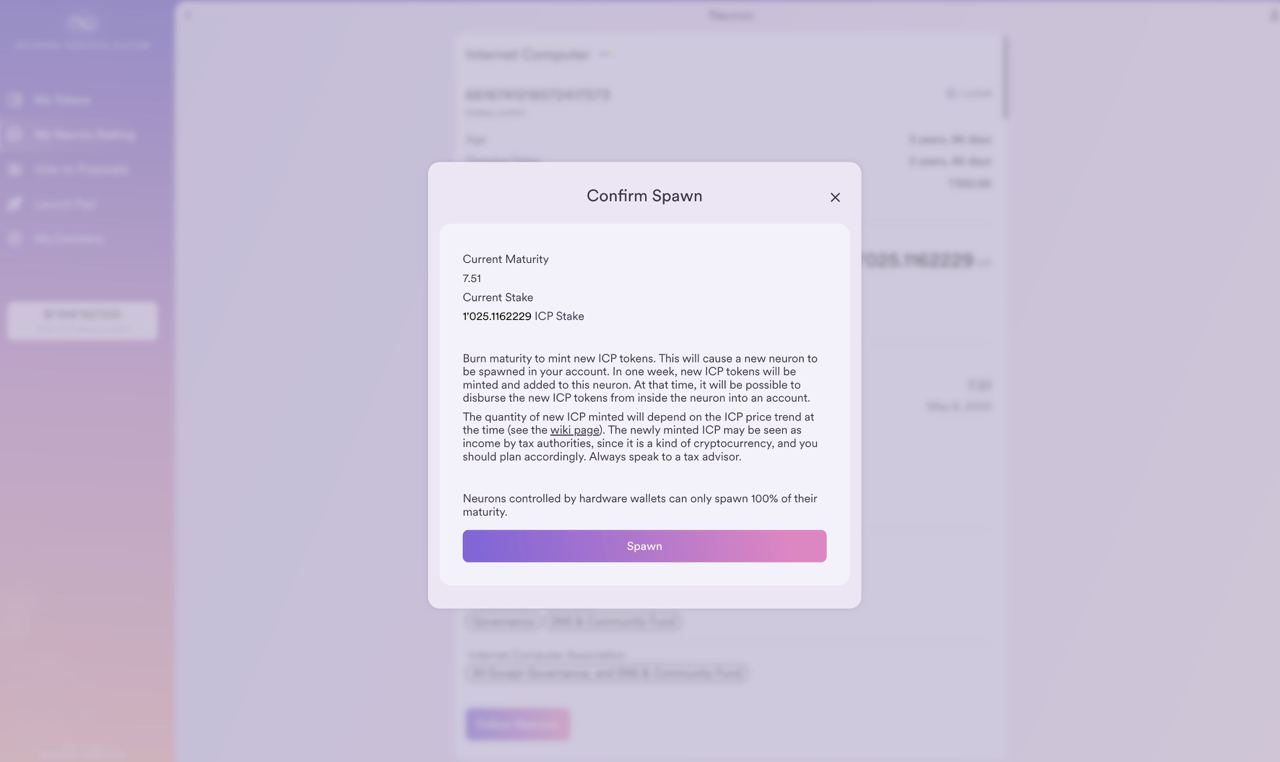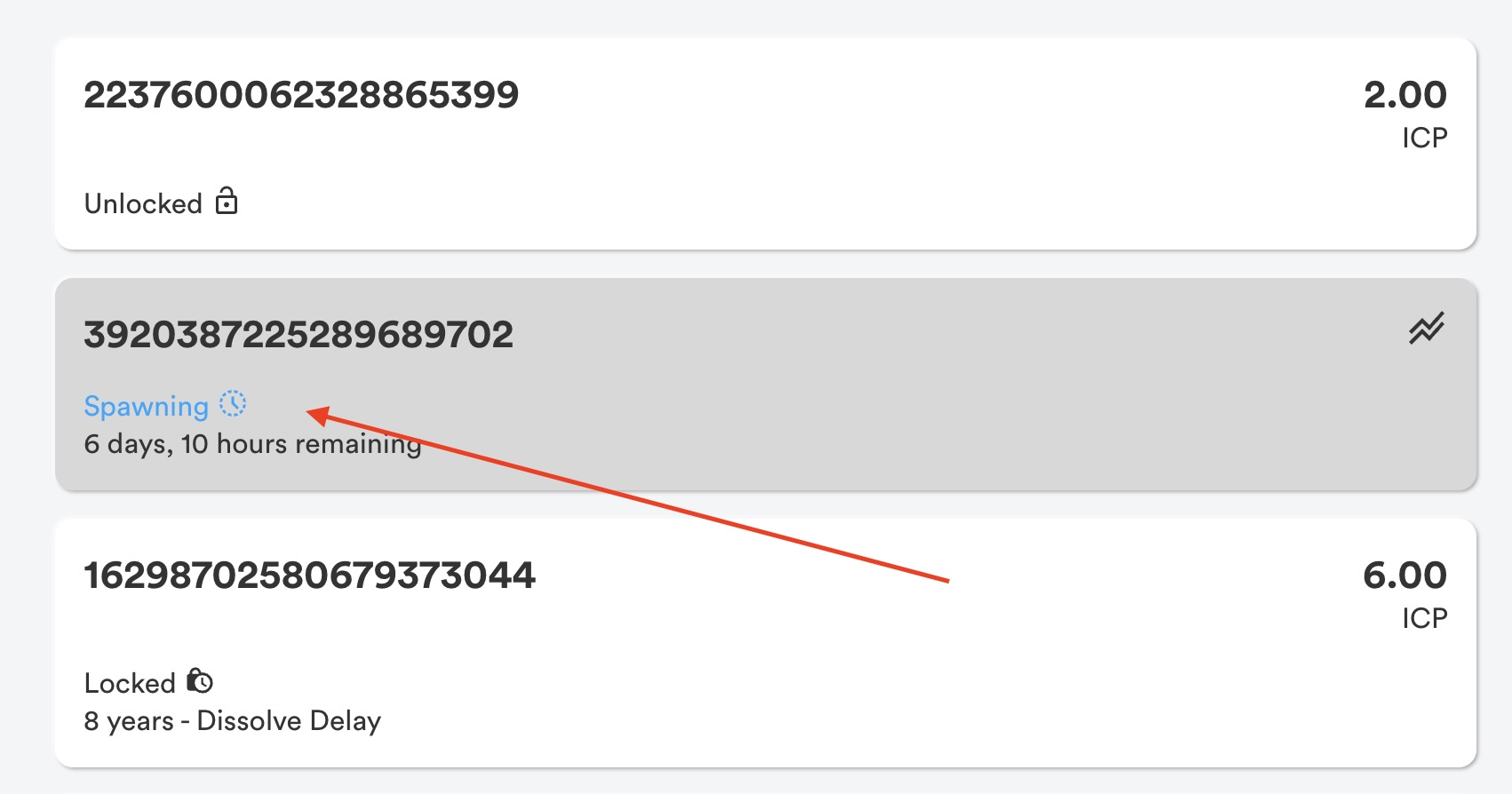# How does maturity modulation work when spawning a new neuron in the NNS dapp?

When you trigger spawn maturity, a new neuron will immediately be spawned. It's important to note that the newly spawned neuron will not have ICP, only maturity.Spawned neurons will have a dissolve delay of 7 days and will be initially set to dissolving. After 7 days, the new neuron will be unlocked and ready to be disbursed. The amount of ICP that will be available and minted from the neuron's maturity will be up to +/- 5% based on the recent market price of ICP.To determine the maturity amount:

1. First, determine the 30-day moving average ICP/Cycles conversion rate, which is displayed on the Internet Computer Dashboard. Label them a1 through a29, where a1 represents the average rate on the previous day, a2 represents the rate two days ago and so on. The 30-day moving average conversion rates are used because they exhibit less variance than day-to-day conversion rates.
2. Then compute the relative 7-day return for each of the last four weeks.
• w1 = (a1 - a8) / a8
• w2 = (a8 - a15) / a15
• w3 = (a15 - a22) / a22
• w4 = (a22 - a29) / a29
3. The values w1, w2, w3, and w4 are bounded from -0.05 to 0.05 by clipping values to the limits of this range. So you're capping by 0.05 and flooring by -0.05.
4. Take the average w = (w1 + w2 + w3 + w4) / 4.
5. The resulting value w is a number between -5% and 5% that determines modulation.
6. The maturity amount x is converted to x * (1 + w) units of ICP.

The maturity modulation function is updated once a day.

Here's an example of the function:

• On Feb 1, 2022, the modulation function is 0.73% which is the average of the relative weekly returns w1 = -4.59%, w2 =-0.63%, w3=5.00%, w4 = 3.13%.
• If a user disburses on the day of transfer at 100 maturity, then this will result in 100.73 ICP.

The maturity modulation process introduces a certain amount of uncertainty with the conversion from maturity to ICP. However, the uncertainty is limited in two ways:

• The modulation value w is between -5% and +5%. This implies that, for example, 100 maturity will be converted into an ICP amount in the range of 95 and 105. This kind of volatility is well in the range of daily conversion rate fluctuations of ICP.
• The modulation value w is calculated at the day of modulation as w = (w1 + w2+ w3 + w4) / 4. At the day of initiation seven days prior, the user can already determine (w2 + w3 + w4) / 4. The missing value w1 can affect the modulation value at most by plus or minus 1.25% (5%/4).
• The modulation value can change at most by 2.5% from one week to the next, as one week drops out of the calculation window and as another week enters.
• The modulation function gives an incentive for users to disburse maturity when the ICP/Cycles conversion rate has been increasing over time. For example, if the ICP/Cycles conversion rate was monotonically increasing, then w1, w2, w3, w4 would be positive along with w, which would incentive users to convert a maturity amount x to x * (1 + w) units of ICP.

The maturity modulation process was created to improve the tax situation for neuron holders, giving them a better idea of how much ICP will result from maturity and making it easier to compound maturity with the least manual effort.

For more information, check out the Internet Computer Wiki and the DFINITY Forum.

Updated

<% if (previousArticle || nextArticle) { %>
<% if (previousArticle) { %>
<% if (previousTitle) { %>

#### <%= previousTitle %>

<% } %> <%= previousArticle.title %>
<% } %> <% if (nextArticle) { %>
<% if (nextTitle) { %>

#### <%= nextTitle %>

<% } %> <%= nextArticle.title %>
<% } %>
<% } %>